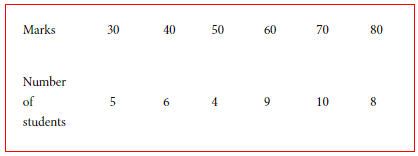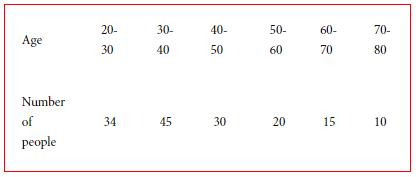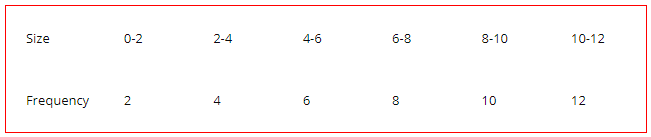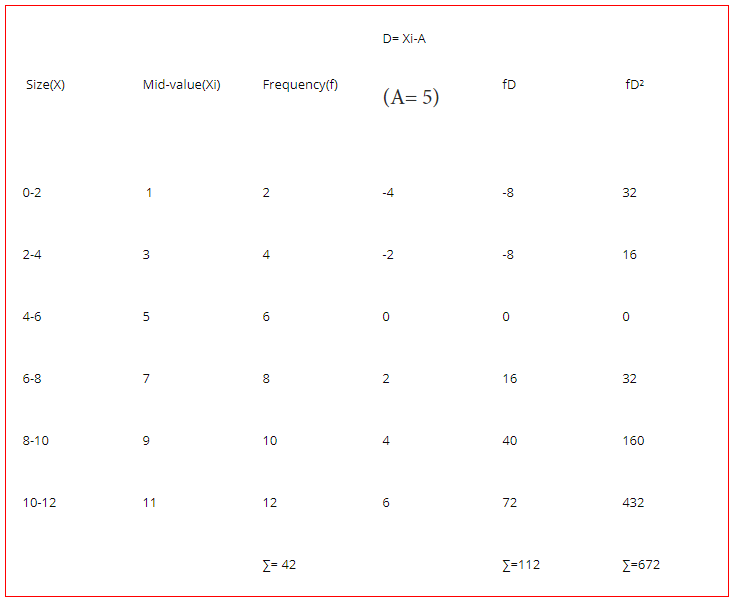# Standard Deviation: Meaning, Concepts, Formulas and Solved Examples

The compilation of these Measures of Dispersion Notes makes students exam preparation simpler and organised.

## Standard Deviation

Suppose you have a certain sum of money that you’re looking to invest. What will be the governing factors to finalize your investment? The risk involved along with the lowest and highest returns will certainly be one if not all. When you focus on the range of returns, you are essentially checking the deviation of returns from the ideal value reflected in advertisements. These factors are a part of a broader concept known as standard deviation.

### Standard Deviation

In statistical analysis, the standard deviation is considered to be a powerful tool to measure dispersion. Effectively dispersion means the value by which items differ from a certain item, in this case, arithmetic mean. Hence, the standard deviation is extensively used to measure deviation and is preferred over other measures of dispersion.

In essence, it is the square root of the arithmetic mean of the squares of deviations of observations from their mean value. It is generally denoted by sigma i.e. σ. There are three characteristic features of standard deviation. Firstly, it is measured by arithmetic mean. In other words, the deviation is calculated taking mean as the reference.

Secondly, it involves dealing with positive values. Unlike mean deviation, where we assume all deviations to be positive, in standard deviation these values are squared. Consequently, there is no such unnecessary assumption made in the case of standard deviation. Lastly, as can be seen from the definition, it is a square root which means its value is always positive.

If we critically analyze standard deviation we can conclude that it is among the best-known measures of dispersion. It is accurate, does not tend to vary a lot with a change in values, and is easy to understand. However, the standard deviation is not free of limitations which include arguments like the difficulty in calculations and extreme observations can tend to affect its value to a large extent. But, considering all the pros and cons, it still wins the race among all measures of dispersion known.### Calculation of Standard Deviation

Before starting with the various approaches towards the calculation of SD we should know about the three types of distribution of data which are:

1. Individual series: There is only a single column of observations.2. Discrete series: There are two columns of data, one consists of the observations, while the other consists of frequencies corresponding to the observations.3. Frequency distribution: It also has two columns consisting of observations and corresponding frequencies. However, the observations are further classified into classes or intervals.### σ for Individual Series

There exist three methods of calculating SD for individual series which are:

Direct Method
In this method, at first, the arithmetic mean is calculated using the formula- ∑X/N. Then deviations of all observations from this mean value are calculated using D = X – mean. In the next step, these deviations, x, are squared and their summation is divided by the number of observations. Lastly, the square root of the above calculation results in the derivation of standard deviation. The formula is mentioned below:

σ = √[∑D²/N]

Here, D = Deviation of an item relative to mean, calculated as D = X – mean
N = The number of observations

Shortcut Method
This method works on the idea of the assumption of any random value for the calculation of deviation. The value is generally assumed such that it lies around the middle of the range of values because if an extreme value is chosen the deviations would be large and hence it will render calculations tedious. The formula is as follows:

σ = √[(∑D²/N) – (∑D/N)²]

Step-deviation method
The step deviation method is an extension of the shortcut method. Further, it simplifies the shortcut method by selecting a common factor among deviations such that when divided by this factor, all the deviation values get reduced. As a result, this reduction makes the calculation simpler. The common factor is generally referred to as C. The formula is mentioned below:

Standard deviation(σ) = √[(∑D’²/N) – (∑D’/N)²] × C

Here, D’ = Step-deviation of observations relative to an assumed mean, calculated as D’ = (X – A)/C
C = Common factor chosen

Note: Standard deviation for individual series can also be calculated by a formula other than the three mentioned above which is as follows: σ = √[(∑X²/N) – (∑X/N)²]

### σ for Discrete Series

The standard deviation for discrete series can be calculated by approaches stated below:

Direct method
As in discrete series another column of frequency gets added, the formula for calculation of standard deviation using direct approach is altered to incorporate frequency is stated below:

Standard deviation(σ) = √(∑fD²)/N)

The formula for calculation of standard deviation using the short-cut method is mentioned below:

Standard deviation(σ) = √[(∑fD²/N) – (∑fD/N)²]

### σ for Frequency Distribution

The three methods to calculate the standard deviation for frequency distribution series are:

Direct Method
The direct method for calculation of standard deviation for frequency distribution is pretty much the same as for discrete series. The only difference occurs when using the values of observations. The mid values of the classes are derived by dividing the sum of the upper and lower values of the class and this value is used for calculations. The formula is:

Standard deviation(σ)= √(∑fD²)/N)

Here, D = Deviation of an item relative to the mean calculated as, D = Xi – Mean
f = Frequencies corresponding to the observations
N = The summation of frequency

Step-deviation Method
The formula is given below:

Standard deviation(σ)= √[(∑fD’²/N) – (∑fD’/N)²] × C

Here, D’ = Step-deviation of observations relative to an assumed value, calculated as D’ = (Xi – A)/C
N = The summation of frequency
C = Common factor chosen

Example:

Question:
Using the short-cut method, calculate the standard deviation of the following series: